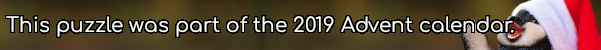mscroggs.co.uk
mscroggs.co.uksubscribeThe sum of the coefficients in the expansion of $$(x+1)^5$$ is 32. Today's number is the sum of the coefficients in the expansion of $$(2x+1)^5$$.